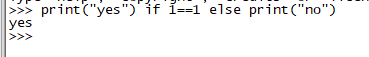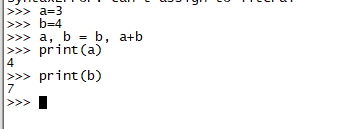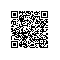# python语法

1.a if b else c

b为真输出a.否则输出c
print("yes") if 1==1 else print("no")2.a, b = b, a+b

a, b = b, a+b 先计算等号右边.再赋值
a=3
b=4
a, b = b, a+b
print(a)
print(b)a=3 b=4 a, b = b, a+b == a,b =4,7 a=3,b=7

3.Python数学运算中*代表乘法，**为指数运算使用钉钉扫一扫加入圈子
+ 订阅